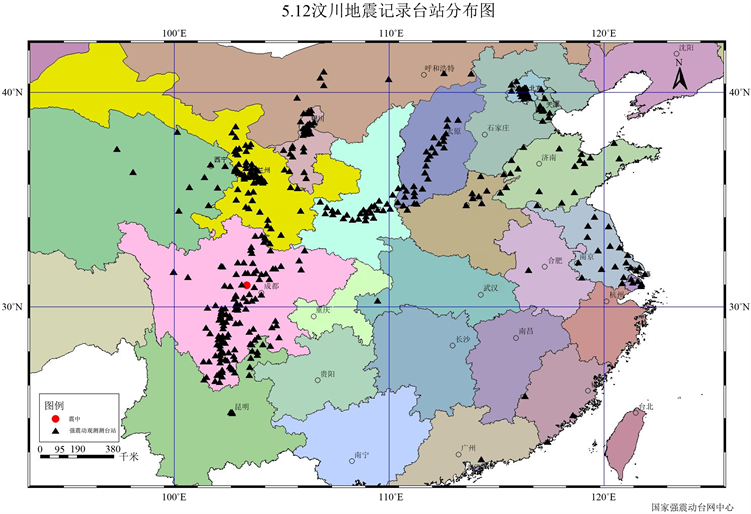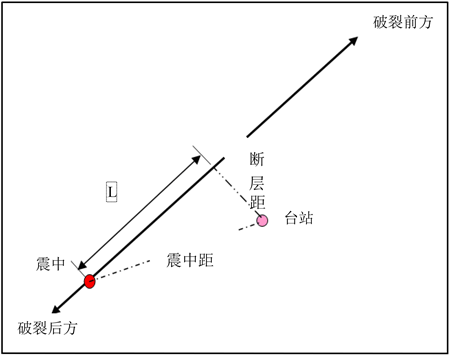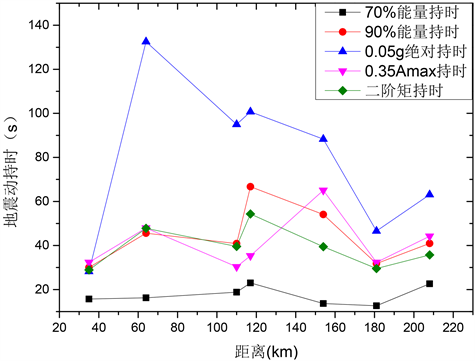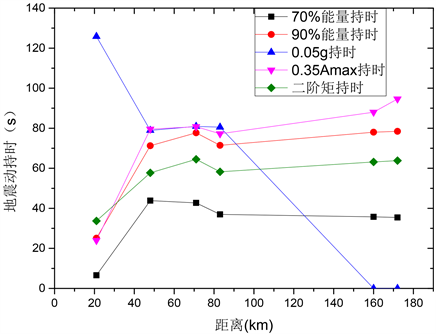﻿ 汶川地震地震动持时特性分析

# 汶川地震地震动持时特性分析Analysis of Ground Motion Duration Characteristics of Wenchuan Earthquake

Abstract: Based on several commonly used definitions of ground motion duration, the statistical results of ground motion duration under different definitions are analyzed and compared by using strong motion acceleration data recorded by China Earthquake Network Center in Wenchuan Earthquake. The results show that the ground motion induced by this earthquake has long duration characteristics, and the statistical results given by different definitions of ground motion duration are quite different. On the other hand, at the same station, absolute strong earthquake duration is the longest and 70% energy duration is the shortest. In addition, using the acceleration records of typical strong motion stations forward and backward rupture, the directional characteristics of ground motion duration are analyzed. The results show that the time duration of ground motion acceleration forward rupture has no obvious trend with the epicenter distance, while the time duration backward rupture increases with the epicenter distance, and the time duration forward rupture is obviously shorter than that behind rupture. The results of this paper can be useful for the study of the influence of the ground motion duration on the seismic response of structures.

1. 引言

2. 地震动持时的定义

1) 绝对持时。又称括号持时，由Bolt  提出，该定义取加速度记录绝对值在第一次和最后一次达到或超过事先设定的值(如0.05 g或0.1 g等)之间所经历的时间作为地震动持续时间。

2) kAmax相对持时。该定义取加速度峰值的k倍作为阈值，强震持时为地震动记录首末两次达到或超过该阈值的时间长度，Amax表示加速度记录峰值，k取0.2~0.5。

3) 能量相对持时。该持时定义最早由Trifunac  提出，常用的有90%能量持时，即将地震动能量达到总能量5%开始至达到总能量的95%结束所经历的时间作为地震动持时。也有用地震动能量达到总能量5%开始至达到总能量的75%结束所经历的时间作为地震动持时，即70%能量持时。如70%能量持时定义公式如下  ：

${T}_{d}={T}_{2}-{T}_{1}$ (1)

$I\left(T\right)=\frac{\underset{0}{\overset{T}{\int }}{a}^{2}\left(t\right)\text{d}t}{\underset{0}{\overset{{T}_{\text{all}}}{\int }}{a}^{2}\left(t\right)\text{d}t},I\left({T}_{1}\right)=0.05,I\left({T}_{2}\right)=0.75$ (2)

4) 均方根持时。如果将地震动加速度时程看作平稳随机过程，该平稳过程的均方根为Arms，持续时间为Td，则满足关系式  ：

$\underset{0}{\overset{T}{\int }}{a}^{2}\left(t\right)\text{d}t={A}_{rms}^{3}{T}_{d}$ (3)

5) 二阶距持时。该定义由谢礼立、周雍年提出  ，该方法记加速度平方图全部面积的重心坐标为Tc，则一般来说(多次地震事件例外)在Tc附近的那个时段应该是地震动时程中震动比较强烈的部分，即主震段或强震段。而平方加速度图上能量分布的二阶距，通过假定在时间Td内加速度峰值出现的平均概率为一次，即可确定持时。

${T}_{d}={|\frac{{\int }_{0}^{T}{\left(t-{T}_{c}\right)}^{2}{a}^{2}\left(t\right)\text{d}t}{{\int }_{0}^{T}{a}^{2}\left(t\right)\text{d}t}|}^{\frac{1}{2}}$ (4)

${T}_{c}=\left({\int }_{0}^{T}t{a}^{2}\left(t\right)\text{d}t\right)/{\int }_{0}^{T}{a}^{2}\left(t\right)\text{d}t$ (5)

${T}_{xz}=2{T}_{d}$ (6)

3. 汶川地震地震动持时统计分析Figure 1. Distribution of Wenchuan earthquake stations (from China earthquake administration)Table 1. Statistical results of ground motion duration with five definitions in the Wenchuan earthquake

4. 地震动持时的方向特性Table 2. Statistical results of ground motion duration in EW direction at forward ruptureTable 3. Statistical results of ground motion duration in EW direction at backward ruptureFigure 2. Diagram of distance LFigure 3. Comparisons of EW direction ground motion duration with distance at forward rupture

1) 不同的定义方法获得的地震动持时结果存在较大差异，一般而言70%能量持时计算获得的结果最小，而0.05 g绝对持时方法计算的持时较大，其他三种定义方法获得的持时变化规律基本一致。

2) 在相近的距离条件下，破裂后方的持时明显大于破裂前方，这是由断层破裂的方向性效应引起的。

3) 断层破裂前方，持时随距离无明显变化规律，如图3所示；而断层破裂的后方，除0.05 g绝对持时方法外，其他四种方法计算的地震动持时随距离增加而不断增加，在超过大约80 km后趋向于稳定。Figure 4. Comparisons of EW direction ground motion duration with distance at backward ruptureFigure 5. Comparisons of ground motion duration with distance in EW direction at forward and backward rupture

4) 在图4中，0.05 g绝对持时在距离超过160 km的泸定水厂与康定均获得了地震动持时为0 s的结果，而实际上两地均在汶川地震中遭受了震害，因此，0.05 g绝对持时方法在本实例中给出的结果明显不合理。

5. 结束语

NOTES

*通讯作者。

 李小军, 周正华, 于海英, 等. 汶川8.0级地震强震动观测及记录初步分析[C]//汶川地震建筑震害调查与灾后重建分析报告, 2008: 1-6.

 周正华, 温瑞智, 王玉石, 等. 地震宏观震害调查的几点建议[J]. 震灾防御技术, 2008, 3(3): 266-270.

 清华大学土木工程结构专家组, 西南交通大学土木工程结构专家组, 北京交通大学土木工程结构专家组, 等. 汶川地震建筑震害分析[J]. 建筑结构学报, 2008, 29(4): 1-9.

 徐锡伟, 闻学泽, 叶建青, 等. 汶川Ms 8.0地震地表破裂带及其发震构造[J]. 地震地质, 2008, 30(3): 597-630.

 卢书楠, 翟长海, 谢礼立. 汶川地震中强震持时的特征研究[J]. 地震工程与工程振动, 2013, 33(2): 1000-1301.

 滕吉文, 白登海, 杨辉, 等. 2008汶川Ms 8.0地震发生的深层过程和动力学响应[J]. 地球物理学报, 2008, 51(5): 1385-1402.

 Bolt, B.A. (1973) Duration of Strong Ground Motion. Proceedings of the 5th World Conference on. Earthquake Engineering, 6, 1304-1313.

 Trifunac, M.D. and Brady, A.G. (1975) A Study on the Duration of Strong Earthquake Ground Motion. Bulletin of the Seismological Society of America, 65, 581-626.

 谢礼立, 张晓志. 地震动记录持时与工程持时[J]. 地震工程与工程振动, 1988, 8(1): 31-38.

 谢礼立, 周雍年. 一个新的地震动持续时间定义[J]. 地震工程与工程振动, 1984, 4(2): 27-35.

 于海英, 王栋, 杨永强, 等. 汶川8.0级地震强震动加速度记录的初步分析[J]. 地震工程与工程振动, 2009, 29(1): 1-13.

Top